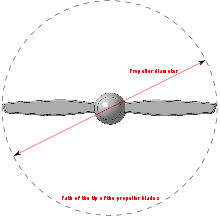﻿Diameter of the propeller.

In aeronautics and marine hydrodynamics, the advance ratio at which a propeller is operating is the ratio between the distance the propeller moves forward through the fluid during one revolution, and the diameter of the propeller. When a propeller-driven vehicle is moving at high speed relative to the fluid the advance ratio of its propeller(s) is a high number; and when it is moving at low speed the advance ratio is a low number. The advance ratio of a propeller is analogous to the angle of attack of an airfoil.

## Contents

The advance ratio J is given by:$J = \frac{V_a}{nD}$

where

 Va is the speed of advance, typically the true airspeed of the aircraft or the water speed of the vessel n is the propeller's rotational speed in revolutions per unit of time D is the propeller's diameter

The advance ratio is a means of describing the incoming angle of the fluid relative to the propeller blade.

## Fluid velocity

The fluid velocity relative to the propeller blade increases with distance from the hub, due to the rotational velocity of the propeller. Therefore, the direction of the flow relative to the propeller blade is equal to the vector sum of the speed of advance and the rotational speed of the propeller. In mathematical terms,$\overrightarrow{Vr} = \overrightarrow{\omega}\times\overrightarrow{r}$$n = \frac{\omega}{(2 \pi)}$$\overrightarrow{V} = \overrightarrow{Va} + \overrightarrow{Vr}$

where

 Vr is the linear velocity vector of the propeller blade at distance r from its center. ω is the propeller's rotational speed in radians per unit of time V is the vector of the incoming fluid flow relative to the propeller blade at distance r from its center r is the distance from the center of the propeller to the radial point of evaluation, 0<=r<=R R is the radius of the propeller

Any propeller, be it for marine or aeronatical use, has blades shaped like airfoils, which twist towards the direction of rotation with increasing distance from the hub. This is to produce a relatively constant "angle of attack" for the blades at the speed of advance for which that particular propeller was designed.

It is worth noting that the formula for the advance ratio is remarkably similar to the ratio of Va/Vr, the difference being a constant of value r/(D*2π), considering that Va and Vr are orthogonal by design, Va/Vr is the tangent of the incoming angle of the fluid relative to the propeller blade. It is for that reason that the advance ratio is in fact analogous to angle of attack for propeller blades.

It is also important to note that the advance ratio J is a non-dimensional parameter, and as such all units on the right side of the equation should cancel out. If the speed of advance is measured in feet per second, for example, the rotational speed must be measured in revolutions per second and the diameter must be measured in feet. The performance of different propellers should be compared for the same advance ratio.

Wikimedia Foundation. 2010.

### Look at other dictionaries:

• advance ratio — The ratio between the distance an aircraft moves from one revolution of a propeller(s), under specified conditions, and the propeller’s diameter. It is the ratio of the forward speed divided by the product of rotational speed and the diameter.… …   Aviation dictionary

• Ratio Studiorum — • The educational system of the Jesuits Catholic Encyclopedia. Kevin Knight. 2006. Ratio Studiorum     Ratio Studiorum     † …   Catholic encyclopedia

• Advance/Decline Ratio- ADR — A market breadth indicator used in technical analysis to compare the number of stocks that closed higher with the number of stocks that closed lower than their previous day s closing prices. To calculate the advance/decline ratio, divide the… …   Investment dictionary

• Advance Rate — (авансовое отношение) отношение первоначального размера займа на приобретение автомобиля к его розничной или оптовой цене. Авансовое отношение эквивалентно отношению размера кредита к стоимости предмета залога (Loan to Value ratio LTV) в жилищном …   Ипотека. Словарь терминов

• diameter ratio — The ratio between the distance an aircraft moves from one revolution of the propeller(s), under specified conditions, and the propeller diameter. Also known as an advance ratio …   Aviation dictionary

• Game Boy Advance — GBA redirects here. For other uses, see GBA (disambiguation). Game Boy Advance …   Wikipedia

• Sonic Advance — Европейская обложка Разработчики Sonic Team Dimps Издатели …   Википедия

• Sonic Advance 2 — Североамериканская обложка Разработчики Sonic Team Dimps Издатели Sega, THQ …   Википедия

• Sonic Advance 3 — Cевероамериканская обложка игры Разработчики Sonic Team Dimps Издатели Sega, THQ …   Википедия

• Tekken Advance — Североамериканская обложка Разработчик Namco Издатель Namco …   Википедия